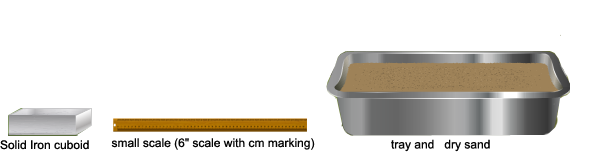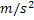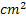Pressure Exerted by a Solid Iron Cuboid on Sand

# Materials required:# The Procedure:

## As performed in a real lab:

Fill ¾ ths of a tray with dry sand and level it.

• Measure the dimensions of a solid iron cuboid accurately using a scale. Mark the three faces of the cuboid as A, B and C.
• Place the solid iron cuboid by the surface A on the plane levelled sand in the tray.
• After a few minutes, remove the Iron cuboid and you will see that it has made a depression in the sand.
• Measure the depth (depression) it has made in the sand using the scale.
• Repeat the same procedure for the other two surfaces.

## As performed using the simulator:

1. You can choose the environment  in which you want to conduct the experiment: Earth or Moon.
2. Select the iron cuboid’s face of your choice by clicking on that particular one.
3. To find the depression made, you’ll first have to remove the iron cuboid from the tray. To do this, just click on the iron cuboid that is in the tray.
4. Now calculate the Area and Pressure and note the values in the respective boxes.  (Area = Length x Breadth) (Pressure = Thrust / Area, where Thrust = Mass x Acceleration due to gravity)
5. Measure the depth (depression) made in the sand using the scale.  To do this, click on the ‘Show Scale’ and ‘Tray Cross Section’ check box to take the measurement.
6. The measurement can be taken by placing the scale against the right cross section of the tray.
7. Repeat the same procedure for the other two surfaces.
8. Once done, the simulation can be repeated by selecting a different environment from the one chosen earlier.

# Observations:

Gravitational force on the environment = ……..1. Calculate the area occupied by each surface of the solid iron cuboid.
• Area occupied by surface A in the sand = .............• Area occupied by surface B in the sand = .............• Area occupied by surface C in the sand = .............1. Calculate the pressure made by each surface of the solid iron cuboid.
• Pressure made by the surface A in the sand = ............. N
• Pressure made by the surface B in the sand = ............. N
• Pressure made by the surface C in the sand = ............. N
1. Calculate the Depression.
• Depression made by the surface A in the sand = ............. cm
• Depression made by the surface B in the sand = ............. cm
• Depression made by the surface C in the sand = ............. cm

# Precautions:

1. Dried sand must be used.
2. The tray must have significant length and width.
3. Appropriate cuboid of dimension must be used.